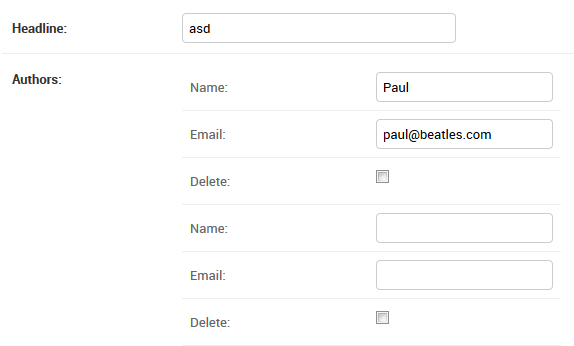# Using Djongo Array Model Field

## ArrayField

With Djongo there can be an array of embedded documents inside the parent document. You can create an embed array/list of models inside the parent model and store it directly into MongoDB.

``````class ArrayField(MongoField):
def __init__(self,
model_container: typing.Type[Model],
model_form_class: typing.Type[forms.ModelForm] = None,
model_form_kwargs: dict = None,
*args, **kwargs):
``````

### Arguments

Argument Type Description
`model_container` `models.Model` The child model class type (not the instance) that this array field will contain.
`model_form_class` `models.forms.ModelForm` The child model form class type of the array model. All child models inside the array must be of the same type. Mixing different types of child models inside the embedded array is not supported.
`model_form_kwargs` `dict()` The kwargs (if any) that must be passed to the `forms.ModelForm` while instantiating it.

### Example

``````from djongo import models
from django import forms

class Blog(models.Model):
name = models.CharField(max_length=100)
tagline = models.TextField()

class Meta:
abstract = True

class BlogForm(forms.ModelForm):
class Meta:
model = Blog
fields = (
'name', 'tagline'
)

class Author(models.Model):
name = models.CharField(max_length=200)
email = models.EmailField()

class Meta:
abstract = True

class AuthorForm(forms.ModelForm):
class Meta:
model = Author
fields = (
'name', 'email'
)

class Entry(models.Model):
blog = models.EmbeddedField(
model_container=Blog,
model_form_class=BlogForm
)

authors = models.ArrayField(
model_container=Author,
model_form_class=AuthorForm
)

objects = models.DjongoManager()
``````

### Creating Array fields

A Model with an Array field can be created as follows:

``````entry = Entry()
entry.authors = [{'name': 'John', 'email': 'john@mail.com'},
{'name': 'Paul', 'email': 'paul@mail.com'}]
entry.save()
``````

### Querying Array fields

Djongo uses a mixture of Django query syntax and MongoDB query syntax. Consider a query to retrieve all entries made by the author Paul. Using `ManyToManyField` this requires 2 SQL queries. First selects the `id` for author Paul from the `author` table. Next, a JOIN with `entry_authors` and `entry` gives the corresponding entries.

With `ArrayField` the query reduces to a single simple query:

``````entries = Entry.objects.filter(authors={'name': 'Paul'})
``````

Djongo lets you get even more specific with your queries. To query all entries where the third author is Paul:

``````entries = Entry.objects.filter(authors={'2.name': 'Paul'})
``````

Note: In MongoDB the first element in the array starts at index 0.

## Using ArrayField in Django Admin

The official Django documentation exemplifies 3 models that interact with each other: Blog, Author and Entry. This tutorial considers the same 3 models. The `blog`; `ForeignKey` of the `Entry` model was optimized in the other tutorial, here we optimize the `authors`; `ManyToManyField`.

``````from djongo import models

class Blog(models.Model):
name = models.CharField(max_length=100)
tagline = models.TextField()

def __str__(self):
return self.name

class Author(models.Model):
name = models.CharField(max_length=200)
email = models.EmailField()

def __str__(self):
return self.name

class Entry(models.Model):
body_text = models.TextField()
pub_date = models.DateField()
mod_date = models.DateField()
authors = models.ManyToManyField(Author)
n_pingbacks = models.IntegerField()
rating = models.IntegerField()

def __str__(self):
``````

A `ManyToManyField` defines a relation wherein an entry is made by several authors. It also defines a relation wherein an author could have made several entries. Django handles this internally by creating another table, the `entry_authors` table which contains different mappings between `entry_id` and `author_id`.

Fetching an entry will require 2 SQL queries. The second query will be an expensive JOIN query across `entry_authors` and `authors`. The Model described above will work perfectly well on MongoDB as well, when you use Djongo as the connector. MongoDB however offers much more powerful ways to make such queries. These queries come at the cost of higher disk space utilization.

As a designer using Djongo, you have the freedom to continue with the above schema. Alternatively, you can define a schema having a trade off on disk space for higher performance.

Let us redefine the `authors` in the `Entry` models using the `ArrayField`:

``````from djongo import models

class Blog(models.Model):
name = models.CharField(max_length=100)
tagline = models.TextField()

class Meta:
abstract = True

pub_date = models.DateField()
mod_date = models.DateField()
n_pingbacks = models.IntegerField()
rating = models.IntegerField()

class Meta:
abstract = True

class Author(models.Model):
name = models.CharField(max_length=200)
email = models.EmailField()

class Meta:
abstract = True

def __str__(self):
return self.name

class Entry(models.Model):
blog = models.EmbeddedField(
model_container=Blog,
)
meta_data = models.EmbeddedField(
)

body_text = models.TextField()

authors = models.ArrayField(
model_container=Author,
)
Notice how the `ManyToManyField` is now replaced by the `ArrayField`. To display the Array field in Django Admin, a `Form` for the field must be present. Since the array is made up of abstract `Author` models, the form can be easily created by using a `ModelForm`. If you do not specify a `ModelForm` for your array models in the `model_form_class` argument, Djongo will automatically generate a `ModelForm` for you.Django Admin reveals multiple neatly nested `Name` and `Email` fields under a single Author label.